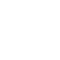# Do you know what is periodic motion, defined with examples?

The motion of a body that repeats in equal intervals of time is known as a periodic motion of the body. In such a motion the said body is to have undergone one cycle after passing through a series of positions before returning to its original state.

## Periodic motion definition;

The motion of a body that repeats in equal intervals of time is known as a periodic motion of the body. In such a motion the said body is to have undergone one cycle after passing through a series of positions before returning to its original state.

We may take an example of the motion of a pendulum to understand it better. If the motion of the pendulum is observed then one can understand that the pendulum passes through the mean position after definite intervals of time. In such a case the interval of time for repetition is known as the cycle of the motion is called a period, whereas the number of periods per unit is known as frequency. The frequency is normally expressed in hertz units, and one hertz is equal to one cycle per second written as Hz. The properties of periodic motion are velocity, period and amplitude.

#### The cause of periodic motion;

The principal cause of periodic motion is the law of inertia. The law of inertia says, that an object shall remain at rest or in motion in a straight line with constant speed unless it is interfered with by a force. The law of inertia is also called Neuton’s first law of motion.

Periodic motion is represented by the following figure-

### Simple harmonic motion;

Simple harmonic motion is treated as a special type of periodic motion and a type of periodic oscillation. In an oscillatory motion, the body moves to and fro about a fixed position where the acceleration is directly proportional to the displacement. The beating of the human heart, vibrating atoms, water waves etc are examples of oscillation.

#### The formula of simple harmonic motion;

The equation of the simple harmonic motion is written as;

x(t)=A cos(ωt+ɸ), where t is time measured in seconds, ω is the angular frequency with units of inverse seconds, A is the amplitude measured in meters or centimetres and ɸ is the phase shift measured in radians(denoted by the symbol rad is unit of angle in SI unit)

The simple harmonic motion has served as a base and mathematical model to define and study other periodic motions for centuries.

## The formula of Periodic motion;

The periodic motion formula represents time period and frequency. The time period is the time taken by the motion to repeat itself and the frequency is the number of times the motion is repeated per second. Let us take the example of the hands of the clock for a better understanding of the above fact. The period of motion of an hour’s hand is twelve hours, whereas, in the case of a minute hand, it is 60 minutes or one hour and of the second hand it is one minute.

Hence the frequency f=1/period=1/time=1/T. However, in the case of a wave, that has velocity v and a wavelength λ, then the period T is equal to λ/v or T=λ/v. Now, f=1/T=v/ λ.

SI unit of frequency is hertz(Hz) and is defined as one cycle per second, i.e. 1Hz=1/s=1s-1

## Some more examples;

• The revolution of the earth and other planets in their respective orbits around the Sun.
• A clock’s pendulum.
• A rocking chair.
• A boy swinging on a swing.
• A vibrating tuning fork.
• A string of a violin.
• The revolution of the moon around the earth.
• The rotation of an electron around a nucleus.

## Non-periodic motion;

When periodic motion is being discussed non-reference of non-periodic motion is definitely incomplete. In a non-periodic type of motion, the object repeats its motion, however, the repetition has no fixed intervals or period of time. Some examples of such motion are;

• Movement of particles in the air.
• The tidal waves in the sea.
• The running of batters between the wickets.
• Mechanical bull ride.
• Bouncing ball.

## Conclusion;

The motion of a body that repeats in equal intervals of time is known as a periodic motion of the body. It is one of the basic principles of physics. During our day to day activities we usually encounter the periodic motion, which reminds us to become regular and sincere.

•February 27, 2023 at 9:40 am
M M Xess

Very nice Topic with simple examples

•